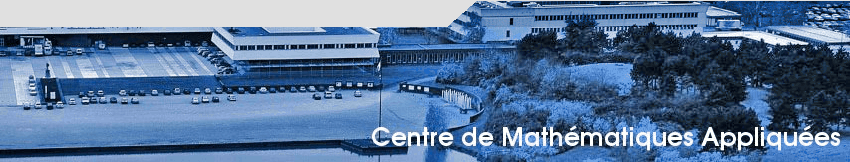• Basics (lecture notes from the MAP431 course at polytechnique - in French) download

• Matlab TP1. Text

• Paper 1.a
• Paper 1.b
• Paper 2
• Paper 3
• Paper 4
• Paper 5

• Comments: The difficulty is evaluated with a number of * (*=easier, ***=difficult). The grading will of course take the difficulty of the subject in consideration.
• ** Papers 1.a and 1.b are about the numerical method called HMM. Here, it would be very desirable to experiment an implementation of the method. Only one paper should be chosen, although it is possible to read both.
• *** Paper 2 is theoretical. It explains the so-called "boundary layer" effect of homogenization with Dirichlet boundary conditions.
• *** Paper 3 is about the numerical method called MSFEM. Again an implementation could be tried. In the paper the accent is put on the error analysis of the method.
• * Paper 4 is the classical method of homogenization (the one seen in TP2) applied to Stokes equations.
• ** Paper 5 is a Gamma-convergence method applied to a problem in magnetism. Theoretical as well.

Good luck!